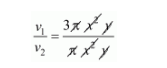# Two cones have their heights in the ratio 1 : 3 and radii 3 : 1.`
Question:

Two cones have their heights in the ratio 1 : 3 and radii 3 : 1. What is the ratio of their volumes?

Solution:

Let the radius of the cone is 3x and x,

And the height of the cone is y and 3y.

Then,

Volume of the first cone

$v_{1}=\frac{1}{3} \pi r^{2} h$

$=\frac{1}{3} \pi(3 x)^{2} y$

$=\frac{1}{3} \pi 9 x^{2} y$

$=3 \pi x^{2} y$.............$(i)$

Volume of the second cone

$v_{2}=\frac{1}{3} \pi(x)^{2} \times 3 y$

$=\pi x^{2} y$............(ii)

Then the radius of their volumeOr

$\frac{v_{1}}{v_{2}}=3: 1$

$v_{1}: v_{2}=3: 1$